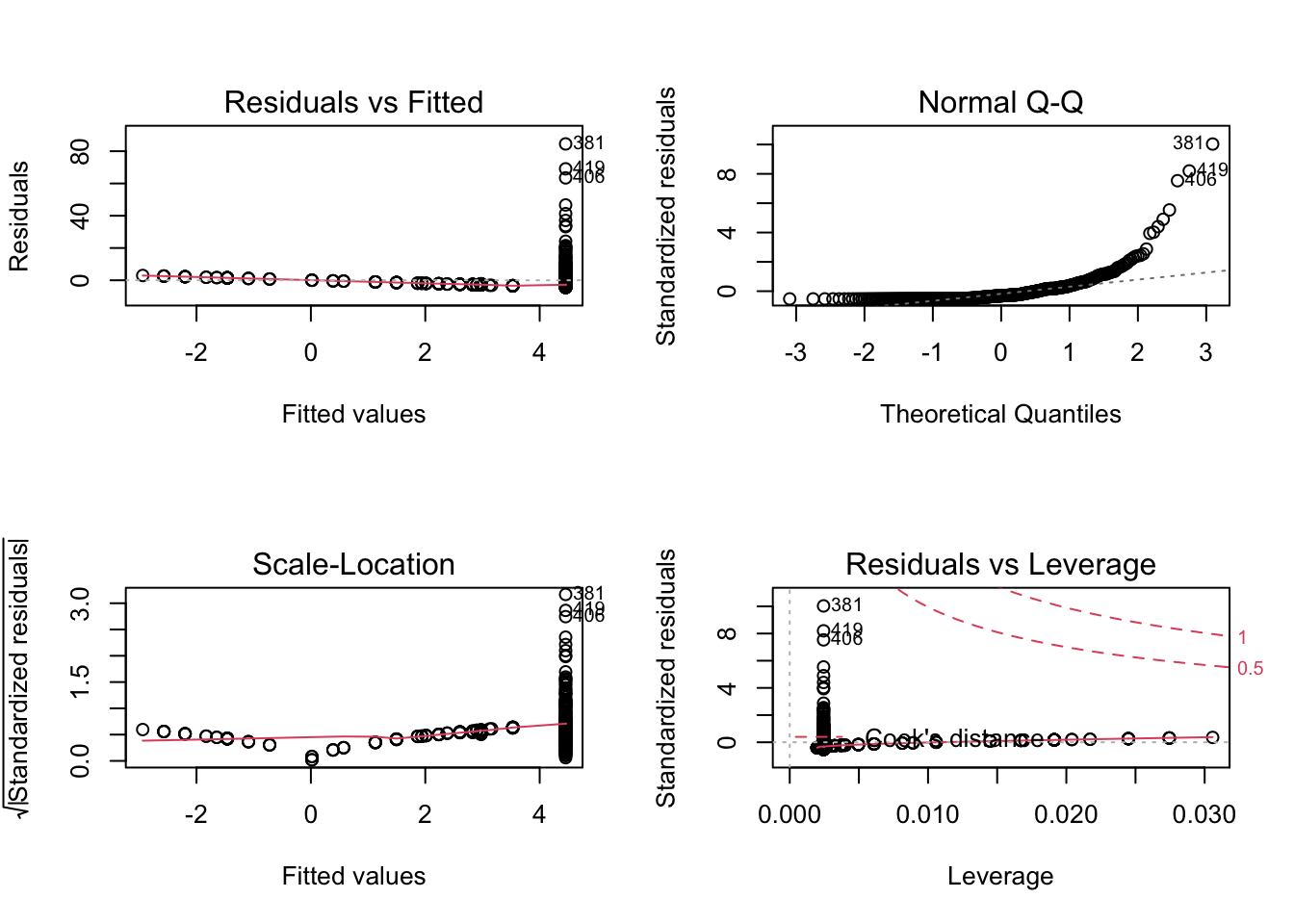ISLR Home

# Question

This problem involves the Boston data set, which we saw in the lab for this chapter. We will now try to predict per capita crime rate using the other variables in this data set. In other words, per capita crime rate is the response, and the other variables are the predictors.

1. For each predictor, fit a simple linear regression model to predict the response. Describe your results. In which of the models is there a statistically significant association between the predictor and the response? Create some plots to back up your assertions.

2. Fit a multiple regression model to predict the response using all of the predictors. Describe your results. For which predictors can we reject the null hypothesis $$H_0 : \beta_j = 0$$?

3. How do your results from (a) compare to your results from (b)? Create a plot displaying the univariate regression coefficients from (a) on the x-axis, and the multiple regression coefficients from (b) on the y-axis. That is, each predictor is displayed as a single point in the plot. Its coefficient in a simple linear regression model is shown on the x-axis, and its coefficient estimate in the multiple linear regression model is shown on the y-axis.

4. Is there evidence of non-linear association between any of the predictors and the response? To answer this question, for each predictor X, fit a model of the form

$Y = \beta_0 + \beta_1X + \beta_2 X^2 + \beta_3 X^3 + \epsilon$

# 15a Simple Linear Regression

Fit each feature one at a time and evaluate

## Model: $$crim = \beta_0 + \beta_1 zn$$

$$crim = \beta_0 + \beta_1 zn$$

### Model Summary

lm.zn = lm(crim ~ zn, data = Boston)
summary(lm.zn)
##
## Call:
## lm(formula = crim ~ zn, data = Boston)
##
## Residuals:
##    Min     1Q Median     3Q    Max
## -4.429 -4.222 -2.620  1.250 84.523
##
## Coefficients:
##             Estimate Std. Error t value Pr(>|t|)
## (Intercept)  4.45369    0.41722  10.675  < 2e-16 ***
## zn          -0.07393    0.01609  -4.594 5.51e-06 ***
## ---
## Signif. codes:  0 '***' 0.001 '**' 0.01 '*' 0.05 '.' 0.1 ' ' 1
##
## Residual standard error: 8.435 on 504 degrees of freedom
## Multiple R-squared:  0.04019,    Adjusted R-squared:  0.03828
## F-statistic:  21.1 on 1 and 504 DF,  p-value: 5.506e-06

Based on the p-value (5.51e-6), zn has a significant association with crim

### Diagnostic Plots

par(mfrow = c(2,2))
plot(lm.zn)library(car)
## Loading required package: carData
##
## Attaching package: 'car'
## The following object is masked from 'package:dplyr':
##
##     recode
## The following object is masked from 'package:purrr':
##
##     some
#qqplot(lm.zn)

## Model: $$crim = \beta_0 + \beta_1 indus$$

$$crim = \beta_0 + \beta_1 indus$$

lm.indus = lm(crim ~ indus, data = Boston)
summary(lm.indus)
##
## Call:
## lm(formula = crim ~ indus, data = Boston)
##
## Residuals:
##     Min      1Q  Median      3Q     Max
## -11.972  -2.698  -0.736   0.712  81.813
##
## Coefficients:
##             Estimate Std. Error t value Pr(>|t|)
## (Intercept) -2.06374    0.66723  -3.093  0.00209 **
## indus        0.50978    0.05102   9.991  < 2e-16 ***
## ---
## Signif. codes:  0 '***' 0.001 '**' 0.01 '*' 0.05 '.' 0.1 ' ' 1
##
## Residual standard error: 7.866 on 504 degrees of freedom
## Multiple R-squared:  0.1653, Adjusted R-squared:  0.1637
## F-statistic: 99.82 on 1 and 504 DF,  p-value: < 2.2e-16

### Diagnostic Plot

par(mfrow = c(2,2))
plot(lm.indus)